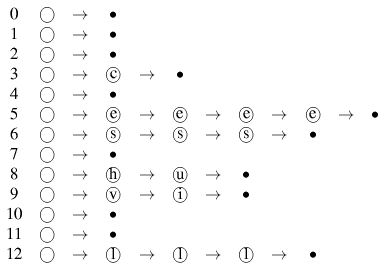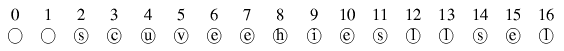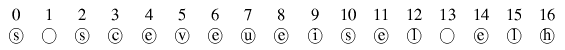# Why do collisions occur when hashing

## Elimination of collisionsThis results in the following table:The greatly simplified example illustrates the method used. Elements that have the same hash value are simply linked to form a list. The numbers in the first row reflect the hash value of the respective list. The circle at the beginning of each list represents the head of the corresponding list, the black point the end of the list, which refers to the implementation.

Code sample
The following code example (Sedgewick) shows how a set of M lists with M list headers can be initialized:Now we have learned a method to avoid collisions. So far so good, but every coin has two sides, including this one. Let us assume that the initial estimate of the effort required for the key to be expected was not very good, or that a large number of collisions occur despite a supposedly good hash procedure. As a result, some of the lists in our table would grow to a size that would severely limit the performance of a sequential search and could only be solved by a complex expansion of the table and redistributing the elements.
An example of a problematic application is the implementation of a telephone book, the data records of which are stored in linked lists by means of hashing. What is the problem? Now, if we look at the distribution of names, we find that (in a larger city there are thousands of surnames that begin with the letter S, but in comparison there are very few that begin with the letters Q, X, or Start Y. As a result, despite a good algorithm, frequent collisions occur, which affect the length of the lists. An antidote would be to increase the available storage space by increasing the number of table spaces M.. The following explanation describes another method of avoiding collisions and making full use of the space in the table.

Linear probing

As described at the beginning, even a very good hash alrogithm can hardly prevent collisions from occurring, even if the number of table spaces available M. is much larger than the number of keys N. Another method to avoid collisions that have arisen is called open addressing. It means that if two keys collide, the colliding element is simply saved in another free space in the table. Would M.=N so each table space would contain exactly one key at the end. But beware: M. must be a prime number! The variant of the open addressingwhich will now be described is called linear probing or linear debugging. To clarify the method of operation, we use our previous example again, with the difference thatinstead ofis. There are only 15 keys, but thereand must be a prime number at the same time, the choice fell on 17.

Example:The following table is created with the help of linear probing:How does this distribution come about?
Basically, the procedure is very simple: The keys calculated above are entered in the table according to their hash values. If a collision occurs, i.e. a table space is already occupied by a key, this is simply mapped to the next free space. This is already the case at the beginning of the table. The keys v and e have the same hash value. v is mapped to position 5 as normal. Should now the key e are mapped, we find that this space in the table is already occupied, so we look for the next free space. This is right next door, i.e. at position 6. The key is now there e filed. The next collision we encounter is with the next e. When it first appeared, place 5 in the table was already occupied, the next free place was at place 6. However, this is already occupied, which is why this key is mapped to table place 7. We go through the places one after the other until a free one is found. When the table ends, the search for a free space begins again at the beginning of the table. The goal, namely the even distribution of the keys, was achieved.

Double hashing

The disadvantage of linear debugging is the often long search time when the table begins to fill. If there are many collisions, all successor positions must be examined accordingly during the search in order to find the correct key, and those that have a completely different hash value must also be examined. However, there is a way to avoid this: Double hashing.
Basically works Double hashing according to the absolutely same principle as that Linear probing. The difference is that a second hash function is used to define a fixed sequence according to which the following table spaces are tested for the key sought. In other words: In the event of a collision, the exact following places are not searched, but a test sequence is established with certain intervals. (Which of course also applies to the mapping of the values ​​on the table.) We remember: The first hash function wasThe second hash function should in this caseread (Sedgewick). The calculationcauses only the last three bits of k be used. Some may now wonder why. Take, for example, the letter R, which occupies the 18th position in the alphabet. Expressed in binary (to five bit positions) this results in 10010. The last three bits of this converted into a decimal value result in the value 2. Subtracted from 8 you get 6, which corresponds to the result that the pocket calculator would also produce if onetyped.

example

Be givenand. Also the keys that we used before.This leads to the following table:This table is also fairly easy to understand if you look at the calculated hash values. The first hash value of each key is used to determine the actual table space, while the second value is used for collision handling. The first collision occurs with the key e because it has the same hash value as before v. In the case of linear probing would e now simply mapped to the next free space. In this case, the second hash value is used to take a certain number of steps in order to use the table space thus determined. In our example, the second value is 3, i.e. e is mapped exactly 3 places further back on the table, i.e. at place 8. If this place were already occupied by another key, a table place that is exactly 3 steps behind would be examined again. If you come across the end of the table, you start the search again from the beginning. The same process is now carried out for all other keys, the first hash value always specifying the table position and the second hash value the step distance that you have to take if this is already occupied.

Next page Up Previous page Contents

Next page:Calculation of the uniform distribution Upwards:Hashing Previous page:Calculation and working method & nbsp content

2002-05-09